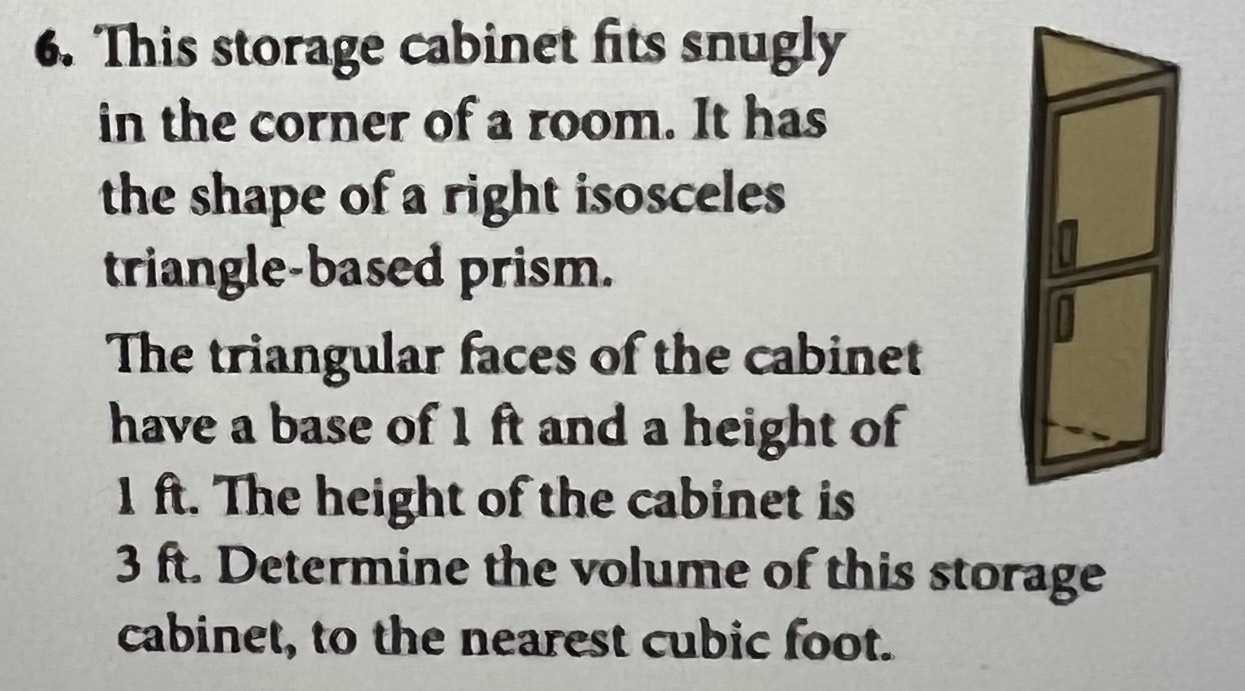### ¿Todavía tienes preguntas de matemáticas?

Pregunte a nuestros tutores expertos
Algebra
Pregunta6. This storage cabinet fits snugly in the corner of a room. It has the shape of a right isosceles triangle-based prism. The triangular faces of the cabinet have a base of $$1 ft$$ and a height of

$$1 ft$$ . The height of the cabinet is $$3 ft$$ . Determine the volume of this storage cabinet, to the nearest cubic foot.

$$V= 1.5ft^3$$